Open in App
Not now

# Class 8 RD Sharma Solutions – Chapter 17 Understanding Shapes Special Types Of Quadrilaterals – Exercise 17.1 | Set 1

• Last Updated : 16 Apr, 2021

### Question 1. Given below is a parallelogram ABCD. Complete each statement along with the definition or property used.

(ii) ∠DCB =

(iii) OC =

(iv) ∠DAB + ∠CDA =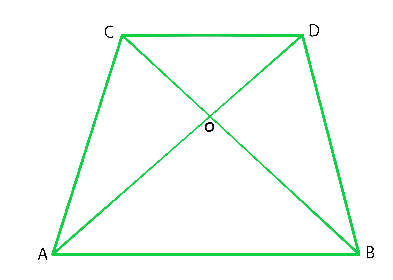Solution:

(i) AD = BC. Because, diagonals bisect each other in a parallelogram.

(ii) ∠DCB = ∠BAD. Because, alternate interior angles are equal.

(iii) OC = OA. Because, diagonals bisect each other in a parallelogram.

(iv) ∠DAB+ ∠CDA = 180°. Because sum of adjacent angles in a parallelogram is 180°.

### Question 2. The following figures are parallelograms. Find the degree values of the unknowns x, y, z.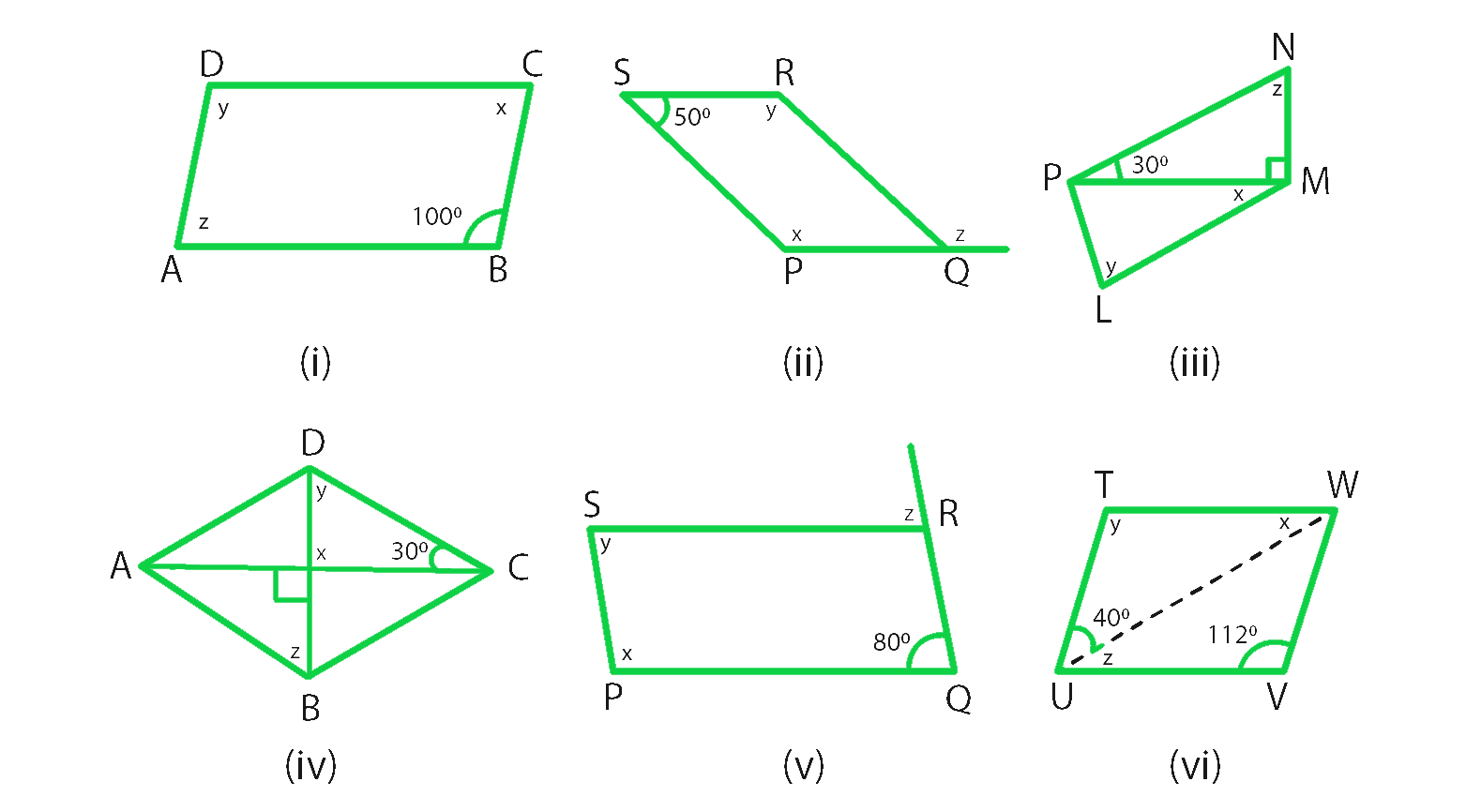Solution:

(i) From figure we conclude that,

∠ABC = ∠y = 100o (Opposite angles are equal in a parallelogram)

∠x + ∠y = 180o (sum of adjacent angles is = 180° in a parallelogram)

∠x + 100° = 180°

∠x = 180° – 100° = 80°

Hence, ∠x = 80° ∠y = 100° ∠z = 80° (opposite angles are equal in a parallelogram)

(ii) From figure we conclude that,

∠RSP + ∠y = 180° (sum of adjacent angles is = 180° in a parallelogram)

∠y + 50° = 180°

∠y = 180° – 50° = 130°

Hence, ∠x = ∠y = 130° (opposite angles are equal in a parallelogram)

From figure, we conclude that,

∠RSP = ∠RQP = 50° (opposite angles are equal in a parallelogram)

∠RQP + ∠z = 180° (linear pair)

50° + ∠z = 180°

∠z = 180° – 50° = 130°

Hence, ∠x = 130°, ∠y = 130° and ∠z = 130°.

(iii) As we know that,

In ΔPMN ∠NPM + ∠NMP + ∠MNP = 180° (Sum of all the angles of a triangle is 180°)

30° + 90° + ∠z = 180°

∠z = 180°-120° = 60°

From figure, we conclude that,

∠y = ∠z = 60° (opposite angles are equal in a parallelogram)

∠z = 180°-120° (sum of the adjacent angles is equal to 180° in a parallelogram)

∠z = 60°

∠z + ∠LMN = 180° (sum of the adjacent angles is equal to 180° in a parallelogram)

60° + 90°+ ∠x = 180°

∠x = 180°-150° = 30°

Hence, ∠x = 30° ∠y = 60° ∠z = 60°

(iv) From figure we conclude that,

∠x = 90° [vertically opposite angles are equal]

In ΔDOC, ∠x + ∠y + 30° = 180° (Sum of all the angles of a triangle is 180°)

90° + 30° + ∠y = 180°

∠y = 180°-120°

∠y = 60°

∠y = ∠z = 60° (alternate interior angles are equal)

Hence, ∠x = 90° ∠y = 60° ∠z = 60°

(v) From figure we conclude that,

∠x + ∠POR = 180° (sum of the adjacent angles is equal to 180° in a parallelogram)

∠x + 80° = 180°

∠x = 180°-80° = 100°

∠y = 80° (opposite angles are equal in a parallelogram)

∠SRQ =∠x = 100°

∠SRQ + ∠z = 180° (Linear pair)

100° + ∠z = 180°

∠z = 180°-100° = 80°

Hence, ∠x = 100°, ∠y = 80° and ∠z = 80°.

(vi) From figure we conclude that,

∠y = 112° (In a parallelogram opposite angles are equal)

∠y + ∠VUT = 180° (In a parallelogram sum of the adjacent angles is equal to 180°)

∠z + 40° + 112° = 180°

∠z = 180°-152° = 28°

∠z =∠x = 28° (alternate interior angles are equal)

Hence, ∠x = 28°, ∠y = 112°, ∠z = 28°.

### Question 3. Can the following figures be parallelograms? Justify your answer.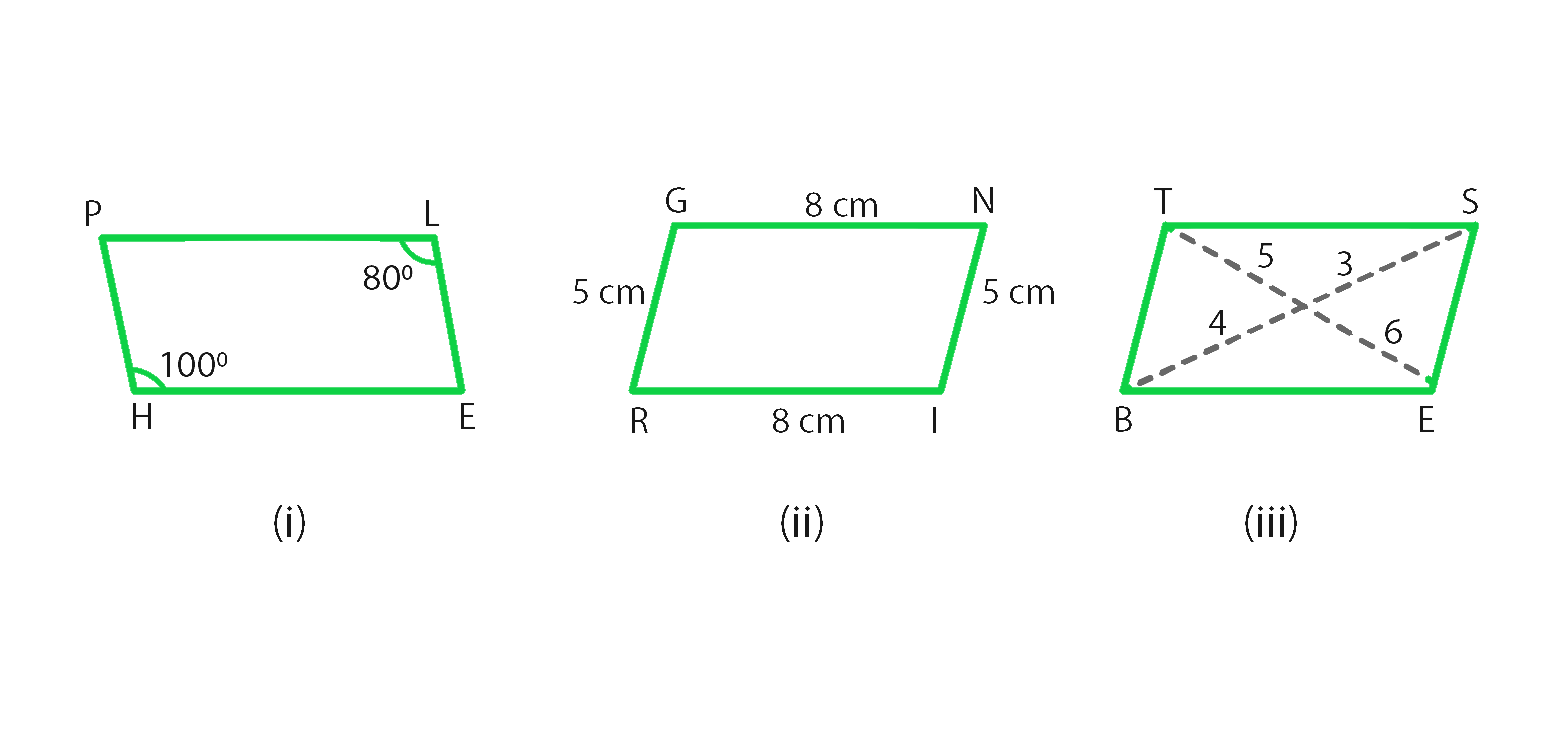Solution:

(i) No, as we know that opposite angles are equal in a parallelogram.

(ii) Yes, as we know that opposite sides are equal and parallel in a parallelogram.

(iii) No, as we know that the diagonals bisect each other in a parallelogram.

### Question 4. In the adjacent figure HOPE is a parallelogram. Find the angle measures x, y, and z. State the geometrical truths you use to find them.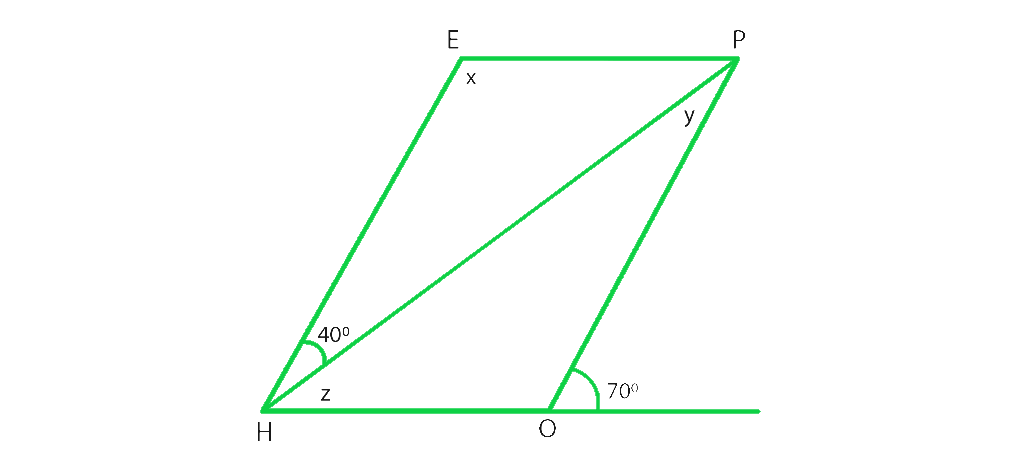Solution:

As we know that,

∠POH + 70° = 180° (Linear pair)

∠POH = 180°-70° = 110°

∠POH = ∠x = 110° (opposite angles are equal in a parallelogram)

∠x + ∠z + 40° = 180° (sum of the adjacent angles is equal to 180° in a parallelogram)

110° + ∠z + 40° = 180°

∠z = 180° – 150° = 30°

∠z +∠y = 70°

∠y + 30° = 70°

∠y = 70°- 30° = 40°

### Question 5. In the following figures, GUNS and RUNS are parallelograms. Find x and y.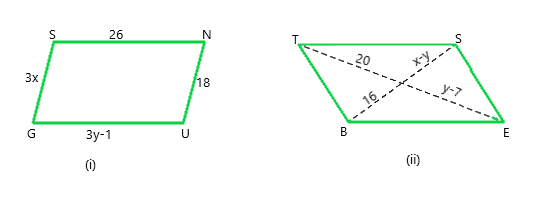Solution:

From figure, we conclude that,

(i) 3y – 1 = 26 (opposite sides are of equal length in a parallelogram)

3y = 26 + 1

y = 27/3 = 9

3x = 18 (opposite sides are of equal length in a parallelogram)

x = 18/3= 6

Hence, x = 6 and y = 9

(ii) y – 7 = 20 (diagonals bisect each other in a parallelogram)

y = 20 + 7 = 27

x – y = 16 (diagonals bisect each other in a parallelogram)

x -27 = 16

x = 16 + 27 = 43

Hence, x = 43 and y = 27

### Question 6. In the following figure RISK and CLUE are parallelograms. Find the measure of x.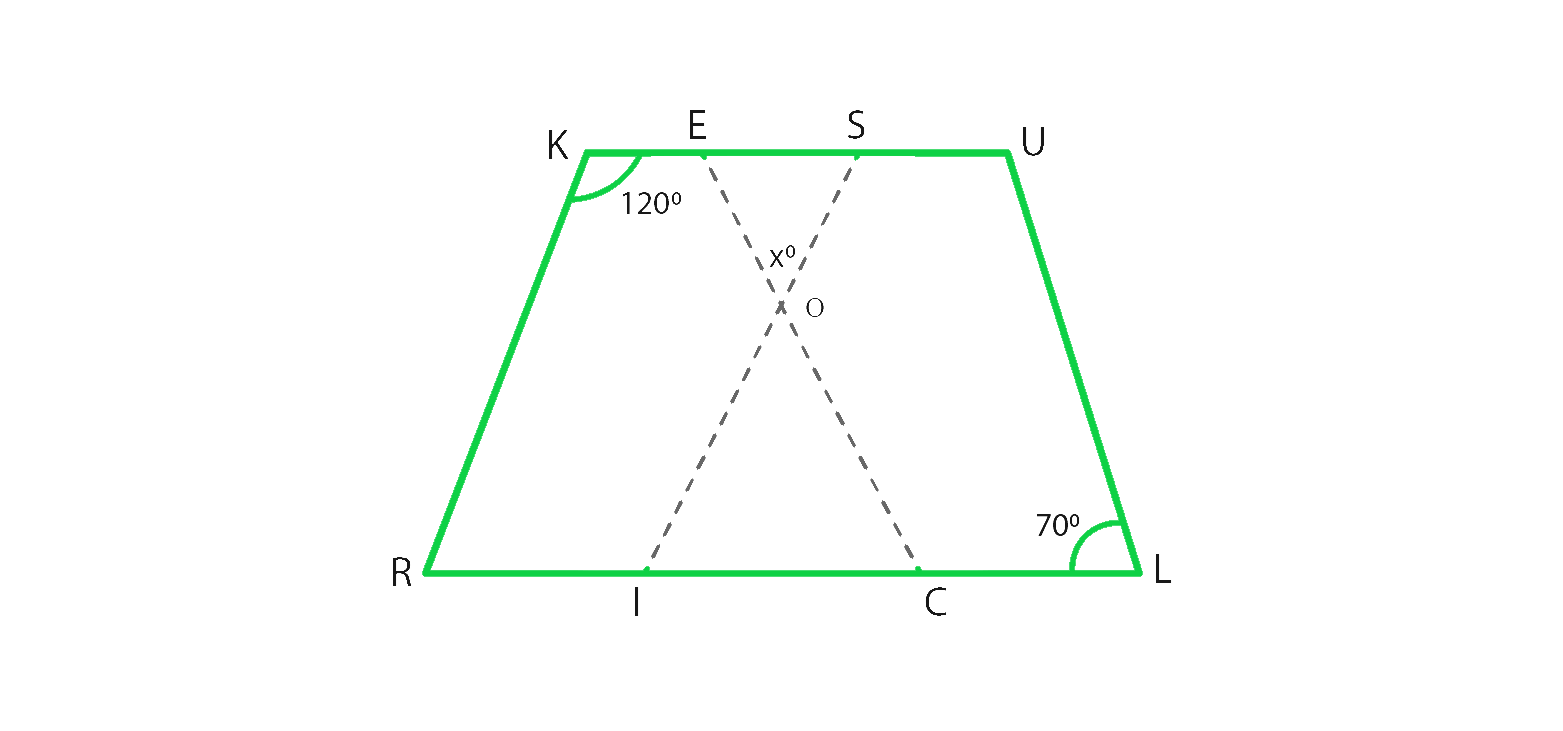Solution:

From figure, we conclude that,

In parallelogram RISK

∠RKS + ∠KSI = 180° (sum of the adjacent angles is equal to 180° in a parallelogram)

120° + ∠KSI = 180°

∠KSI = 180° – 120° = 60°

In parallelogram CLUE,

∠CEU = ∠CLU = 70° (opposite angles are equal in a parallelogram)

In ΔEOS,

70° + ∠x + 60° = 180° (Sum of angles of a triangles is 180°)

∠x = 180° – 130° = 50°

Hence, x = 50°

### Question 7. Two opposite angles of a parallelogram are (3x – 2)° and (50 – x)°. Find the measure of each angle of the parallelogram.

Solution:

As we know that the opposite angles of a parallelogram are equal.

So, (3x – 2)° = (50 – x)°

3xo – 2° = 50° – x°

3x° + xo = 50° + 2°

4x° = 52°

xo = 52°/4 = 13°

The opposite angles are,

(3x – 2)° = 3×13 – 2 = 37°

(50 – x)° = 50 – 13 = 37°

As we know that Sum of adjacent angles = 180°

Other two angles are 180° – 37° = 143°

Hence, Measure of each angle is 37°, 143°, 37°, 143°.

### Question 8. If an angle of a parallelogram is two-third of its adjacent angle, find the angles of the parallelogram.

Solution:

Let us assume that one of the adjacent angle as x°,

then other adjacent angle is = 2x°/3

As we know that sum of adjacent angles = 180°

Therefore,

x° + 2x°/3 = 180°

(3x° + 2x°)/3 = 180°

5x°/3 = 180°

x° = 180°×3/5 = 108°

Other angle is = 180° – 108° = 72°

Hence, Angles of a parallelogram are 72°, 72°, 108°, 108°.

### Question 9. The measure of one angle of a parallelogram is 70°. What are the measures of the remaining angles?

Solution:

Let us assume that the one of the adjacent angle as x°

As we know that sum of adjacent angles = 180°

Therefore,

x° + 70° = 180°

x° = 180° – 70° = 110°

Hence, Measures of the remaining angles are 70°, 70°, 110° and 110°

### Question 10. Two adjacent angles of a parallelogram are as 1: 2. Find the measures of all the angles of the parallelogram.

Solution:

Let us assume that one of the adjacent angle as x°,

then other adjacent angle = 2x°

As we know that sum of adjacent angles = 180°

Therefore,

x° + 2x° = 180°

3x° = 180°

x° = 180°/3 = 60°

So other angle is 2x = 2×60 = 120°

Hence, Measures of the remaining angles are 60°, 60°, 120° and 120°

### Question 11. In a parallelogram ABCD, ∠D= 135°, determine the measure of ∠A and ∠B.

Solution:

Given that,

one of the adjacent angle ∠D = 135°

Let us assume that other adjacent angle ∠A be = x°

As we know that sum of adjacent angles = 180°

x° + 135° = 180°

x° = 180° – 135° = 45°

∠A = x° = 45°

As we know that the opposite angles are equal in a parallelogram.

Therefore, ∠A = ∠C = 45°

and ∠D = ∠B = 135°.

### Question 12. ABCD is a parallelogram in which ∠A = 70°. Compute ∠B, ∠C, and ∠D.

Solution:

Given that,

one of the adjacent angle ∠A = 70°

and other adjacent angle ∠B is = x°

As we know that sum of adjacent angles = 180°

x° + 70° = 180°

x° = 180° – 70° = 110°

∠B = x° = 110°

As we know that the opposite angles are equal in a parallelogram.

Therefore, ∠A = ∠C = 70°

and ∠D = ∠B = 110°

### Question 13. The sum of two opposite angles of a parallelogram is 130°. Find all the angles of the parallelogram.

Solution:

From figure, we conclude that, ABCD is a parallelogram

∠A + ∠C = 130°

Here ∠A and ∠C are opposite angles

Therefore ∠C = 130/2 = 65°

As we know that sum of adjacent angles is 180

∠B + ∠D = 180

65 + ∠D = 180

∠D = 180 – 65 = 115

∠D = ∠B = 115 (Opposite angles)

Hence, ∠A = 65°, ∠B = 115°, ∠C = 65° and ∠D = 115°.

### Question 14. All the angles of a quadrilateral are equal to each other. Find the measure of each. Is the quadrilateral a parallelogram? What special type of parallelogram is it?

Solution:

Let us assume that each angle of a parallelogram as xo

As we know that sum of angles = 360°

x° + x° + x° + x° = 360°

4 x° = 360°

x° = 360°/4 = 90°

Hence, each angle is 90°

Yes, this quadrilateral is a parallelogram.

Since each angle of a parallelogram is equal to 90°, so it is a rectangle.

### Question 15. Two adjacent sides of a parallelogram are 4 cm and 3 cm respectively. Find its perimeter.

Solution:

As we know that opposite sides of a parallelogram are parallel and equal.

therefore, Perimeter = Sum of all sides (there are 4 sides)

Perimeter = 4 + 3 + 4 + 3 = 14 cm

Hence, Perimeter is 14 cm.

### Chapter 17 Understanding Shapes Special Types Of Quadrilaterals – Exercise 17.1 | Set 2

My Personal Notes arrow_drop_up
Related Articles